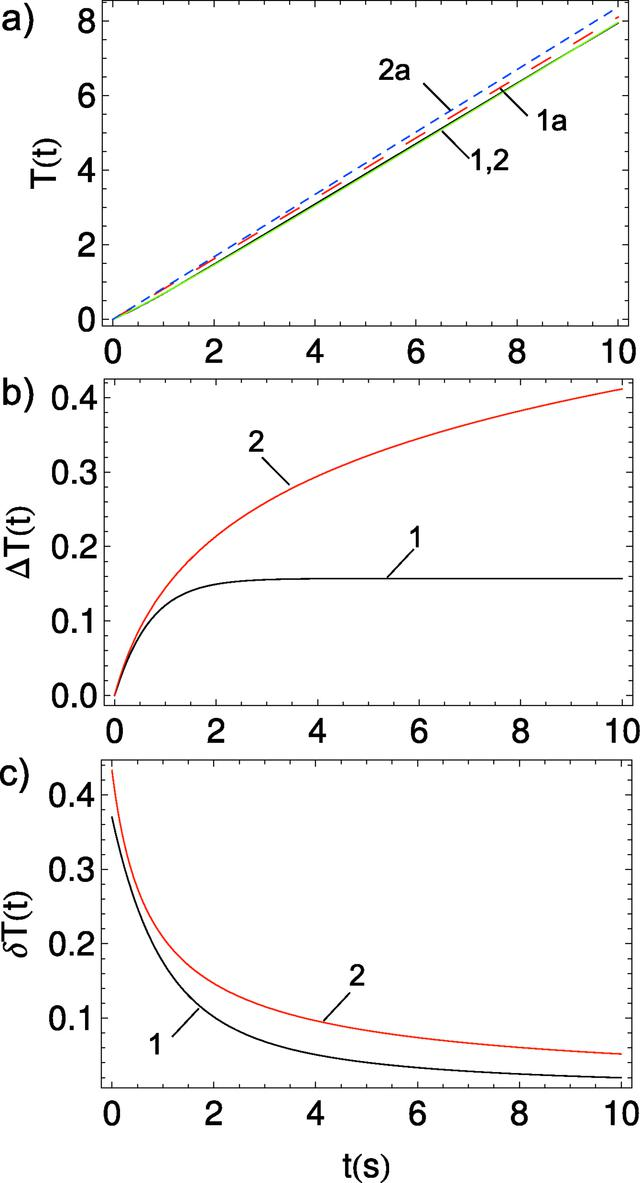disable zoom     view article Figure 9 T(t) integral as a function of exposure time using the main term of the asymptotic expression for the two models of A(t). (a) Exact integration for T(t): model 1 (1, black solid) and model 2 (2, green solid); the asymptotic solution: model 1 (1a, red large dashed) and model 2 (2a, blue small dashed). (b) Absolute T(t) differences of asymptotic and exact solutions for model 1 (black, 1) and model 2 (red, 2), respectively. (c) Relative T(t) difference: the difference presented in (b) divided by the exact solution for model 1 (black, 1) and model 2 (red, 2), respectively.JOURNAL OFSYNCHROTRONRADIATION
ISSN: 1600-5775
Volume 25| Part 3| May 2018| Pages 833-847